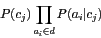DATA MINING
Desktop Survival Guide
by Graham Williams# Algorithm

The basis of naïve Bayes is Bayes theorem. Essentially we want to classify a new record based on probabilities estimated from the training data. That is, we want to determine the probability of a hypothesis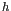(or specifically class membership) given the training data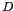(i.e.,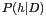) Bayes theorem gives us the clue to calculating this value: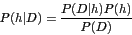Ignoring the detail we can work with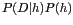to identify a hypothesis that best matches our data (since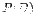is constant for a particular). Both of these quantities we can estimate from the training data.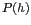is simply the proportion of the database consistent with hypothesis(i.e., the proportion of entities with class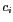--is regarded as the hypothesis).

Calculation of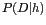still poses some challenges and this is where the naïve part of naïve Bayes comes in. The naïve assumption is that the variables (each recordis describe by variables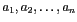) are conditionally independent given the class (i.e., the hypothesis that the classification is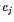, which could be the class soft in our example data) of the record. That is, given that a patient has a soft contact lens, to use our example, the probability of the patient being all of young, myope, non-astigmatic and with a normal-tear-rate is the same as the product of the individual probabilities. The naïve assumption, more concretely, is that being astigmatic or not, for example, does not affect the relationship between being young given the use of soft lenses. Mathematically we write this as: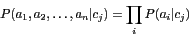Empirically determining the values of the joint probability on the left of this equation is a problem. To estimate it from the data we need to have available in the data every possible combination of values and examples of their classification so we can then use these frequencies to estimate the probabilities. This is usually not feasible. However, the collection of probabilities on the right poses little difficulty. We can easily obtain the estimates of these probabilities by counting their occurrence in the database.

For example, we can count the number of patients with Age being young and belonging to class soft (perhaps there are only two) and divide this by the number of patients overall belonging to class soft (perhaps there are five). The resulting estimate of the probability (i.e.,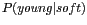) is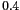.

The Naïve Bayes algorithm is then quite simple. From the training data we estimate the probability of each class,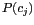, by the proportions exhibited in the database. Similarly the probabilities of each variable's value, given a particular class (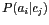), is simply the proportion of those training entities with that class having the particular variable value.

Now to place a new record (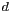) into a class we simply assign it to the class with the highest probability. That is, we choose thewhich maximises: